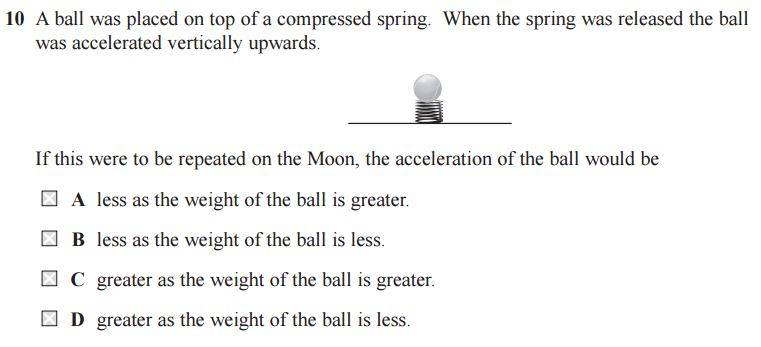# Acceleration on the Moon

## Homework StatementF=ma

## The Attempt at a Solution

I know it's either B or D

Weight = mg so let's say mass of ball is 11kg and gravity on moon is 6, so weight would be 66N

since acceleration a = F/m and we can say that F=mg

therefore a = mg / m which leaves: a=g

and because on the moon the gravity is less this means acceleration is less....yet this is wrong and the answer is D??

Janus
Staff Emeritus
Gold Member
You calculated the acceleration due to gravity, not the acceleration of the ball due to the force of the spring. One acts downward and the other upward.

You calculated the acceleration due to gravity, not the acceleration of the ball due to the force of the spring. One acts downward and the other upward.

Yep, I completely glossed over that, thanks.

Intuitively it makes sense now, but is there a formula I can use to get a better understanding?

jbriggs444